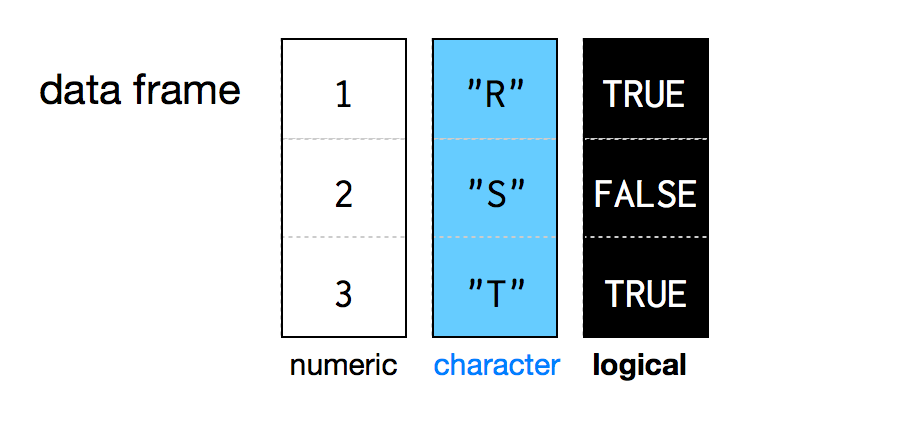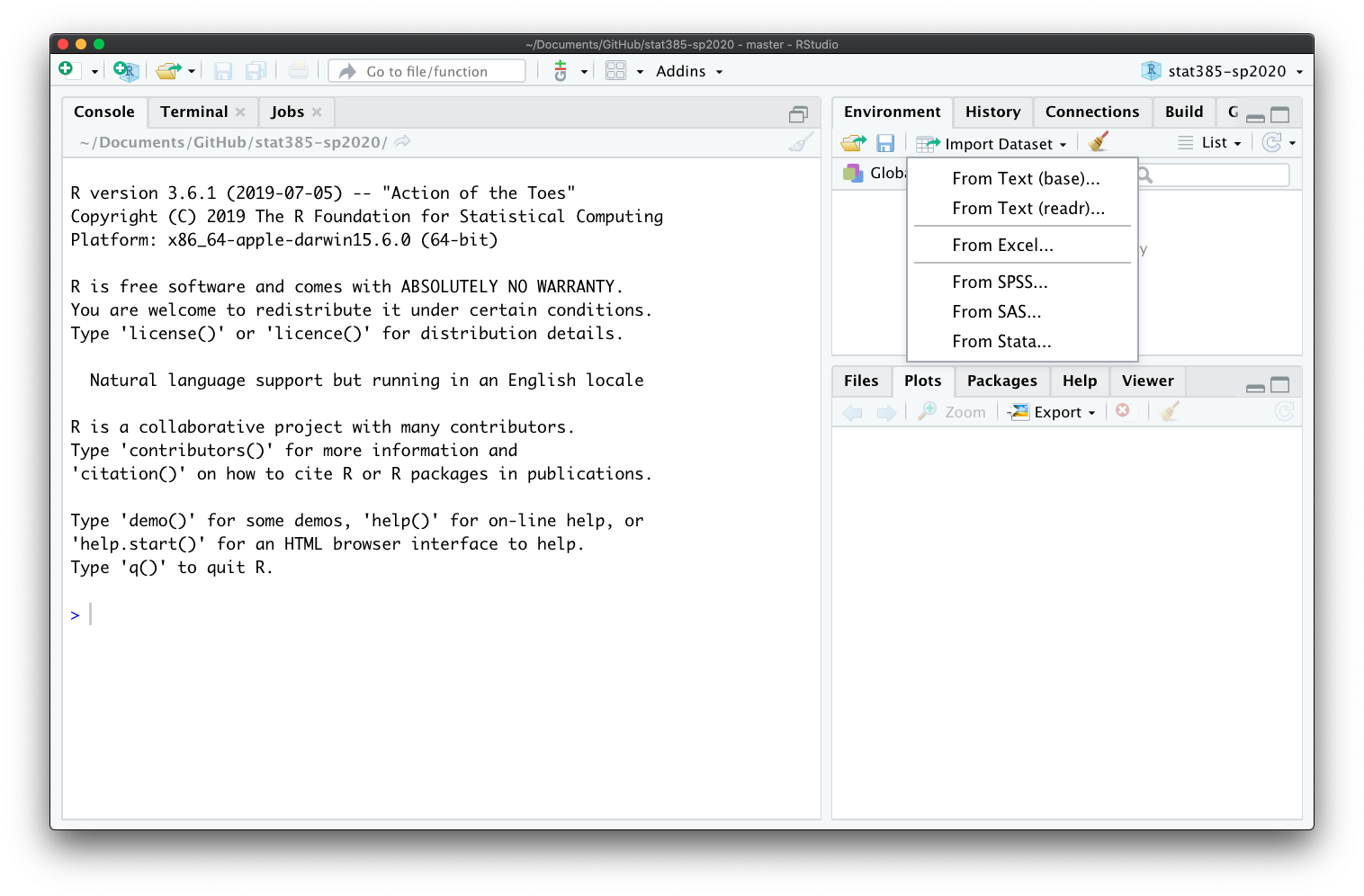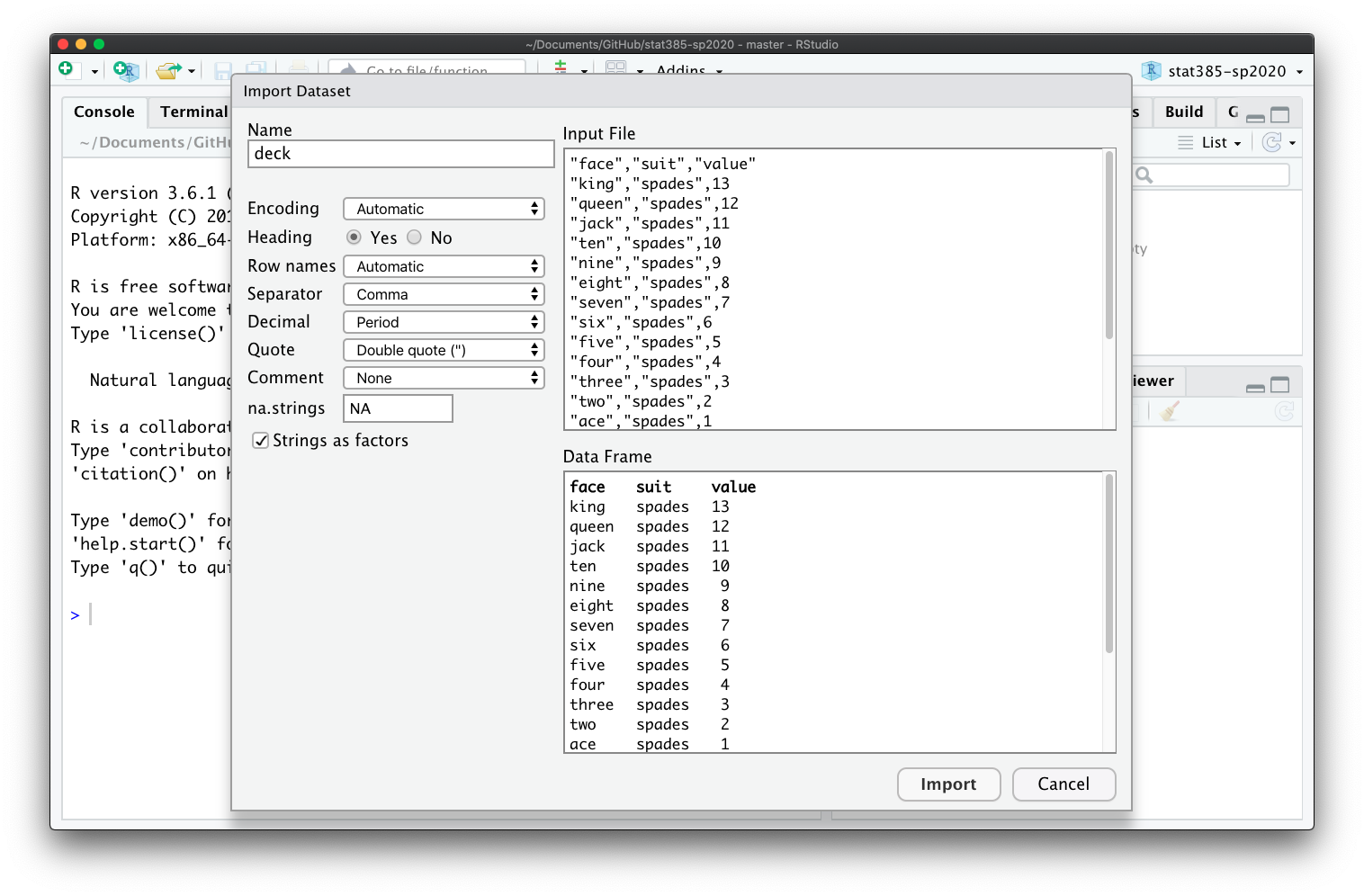# Intro to Data Frames in R

## Review

• Which data structures have we learned so far?
• Vector:
• Atomic Vector
• List
• Matrix
• Array

## Data Frames

• Data frames are the two-dimensional version of a list.
• Store data in a table format, like an Excel spreadsheet.
``faithful``
• Data frames group vectors together into a two-dimensional table.
• Each vector becomes a column in the table. Hence, every column must be of the same length.
• Each column of a data frame can contain a different type of data.
• But within a column, every cell must be of the same type of data.A data frame is a list of vectors of the same length.

### Creating a Data Frame

• Use `data.frame()` function to creata a data frame by hand:
``````cards <- data.frame(face = c("ace", "two", "six"),
suit = c("clubs", "clubs", "clubs"),
value = c(1, 2, 3))
cards``````
• The `str()` function show what types of objects are grouped together in the data frame.
``str(cards)``
``````## 'data.frame':    3 obs. of  3 variables:
##  \$ face : Factor w/ 3 levels "ace","six","two": 1 3 2
##  \$ suit : Factor w/ 1 level "clubs": 1 1 1
##  \$ value: num  1 2 3``````

## Loading Data

### Using RStudio Interface

• Download the file deck.csv to your computer.
• CSV is short for comma-separated values file.
• We will first learn how to load data through the RStudio interface.